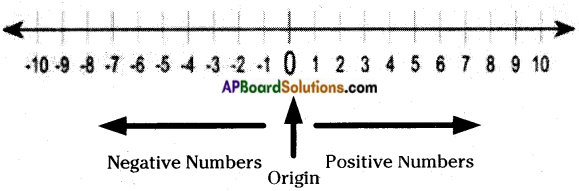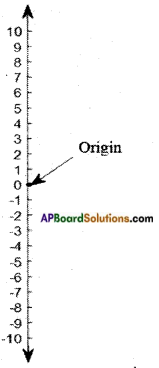Students can go through AP Board 6th Class Maths Notes Chapter 4 Integers to understand and remember the concepts easily.

## AP State Board Syllabus 6th Class Maths Notes Chapter 4 Integers

→ Positive numbers: All numbers which are greater than zero are called positive numbers {1, 2, 3, …..}

→ Negative numbers: In our real life we come across many situations where in we have to use numbers whose value is less than zero; such numbers are called negative numbers.
Example: Very low temperature, loss in a business, depth below a surface, etc. Negative numbers are represented by the minus symbol

→ Zero is neither a negative number nor a positive number.→ Integers: The set of positive numbers, zero along with the set of negative numbers are called Integers. The set of integers is denoted by I or Z.
I = Z = {…. 4, -3, -2, -1, 0, 1, 2, 3,…..}

→ Historical Notes: Brahma Gupta (598 – 670 AD), Indian mathematician first used a special sign (-) for negative numbers and stated rules for operations on negative numbers.
The letter ”Z” was first used by the Germans because the word for Integers in the language is Zehlen which means NUMBER.

→ Representation of Integers on a number line:On a number line all negative numbers lie on the left side of zero. All positive numbers lie on right side of zero.
A number line extends on either side endlessly.
All whole numbers are called non-negative integers.
The natural numbers are called positive integers.On a number, of the given two numbers the number on LHS is always less than the number on the RHS.On a number line as we move from left to right the value of numbers goes on increasing and vice versa.
A number line can be written in a vertical direction as given below.